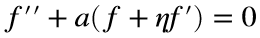# Problem 51625. Solve an ODE: diffusion problem 2

Problem statement
In the solution of a problem involving diffusion, the following ordinary differential equation ariseswhere a is a positive constant and primes denote differentiation with respect to the independent variable η. The function f and its derivatives vanish at infinity, and it is subject to the constraintWrite a function to solve this problem—that is, return values of f at specified values of η.
Background
The physical problem involves diffusion of a quantity instantaneously injected at a point—for example, a spill of a contaminant in an initially clean river. The ODE results from transforming the diffusion equation—a partial differential equation in time and a spatial coordinate—with a similarity solution.

### Solution Stats

83.33% Correct | 16.67% Incorrect
Last Solution submitted on Oct 04, 2023

### Community Treasure Hunt

Find the treasures in MATLAB Central and discover how the community can help you!

Start Hunting!